# RD Sharma Solutions Class 12 Straight Line In Space Exercise 28.3

RD Sharma Solutions for Class 12 Maths Chapter 28 Straight Line in Space Exercise 28.3, is given here for students to excel in their board exams. Solutions that are provided here will help you in getting acquainted with a wide variety of questions and thus, develop problem-solving skills. The pdf of RD Sharma Solutions for Class 12 Maths can be easily downloaded from the links provided below.

## Download PDF of Rd Sharma Solution for Class 12 Maths Chapter 28 Exercise 3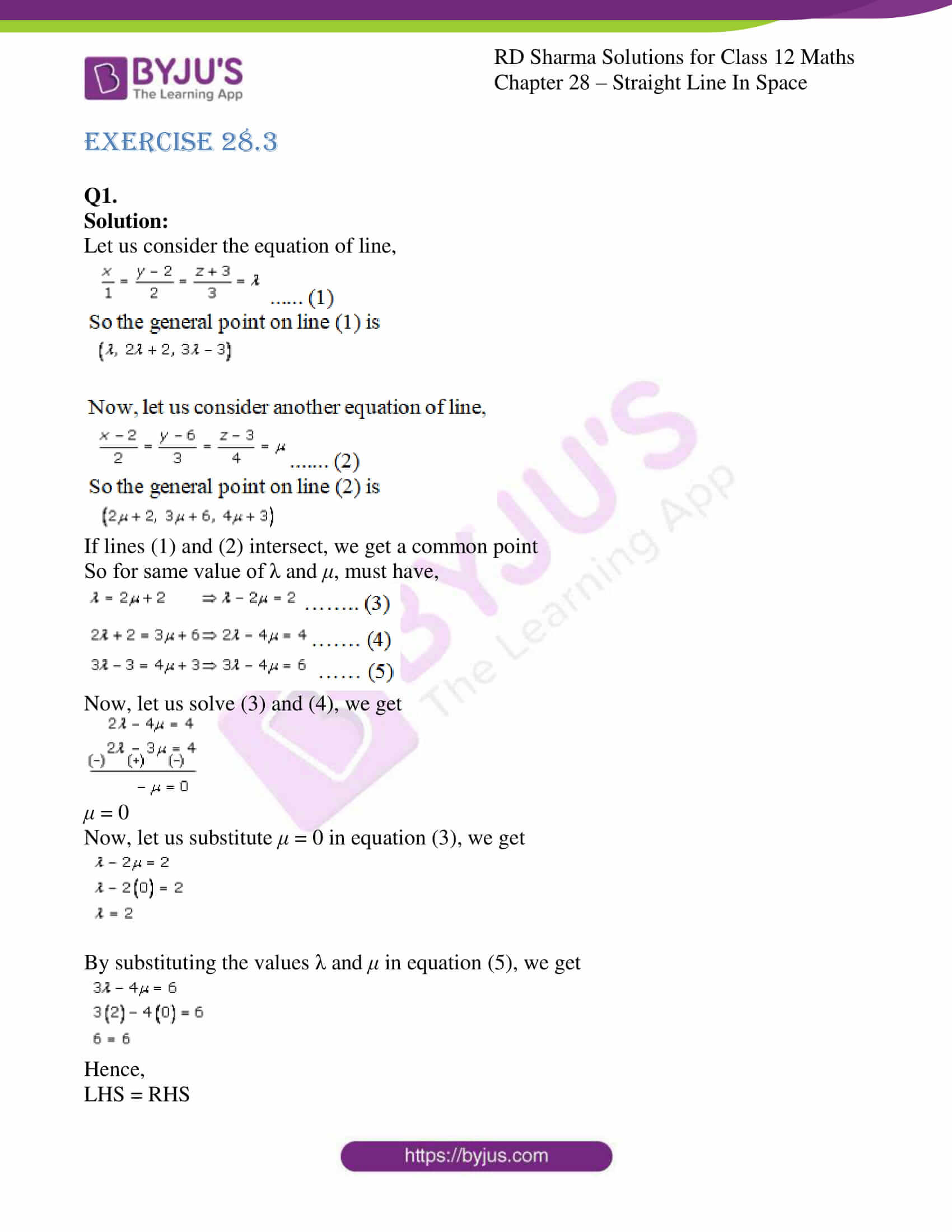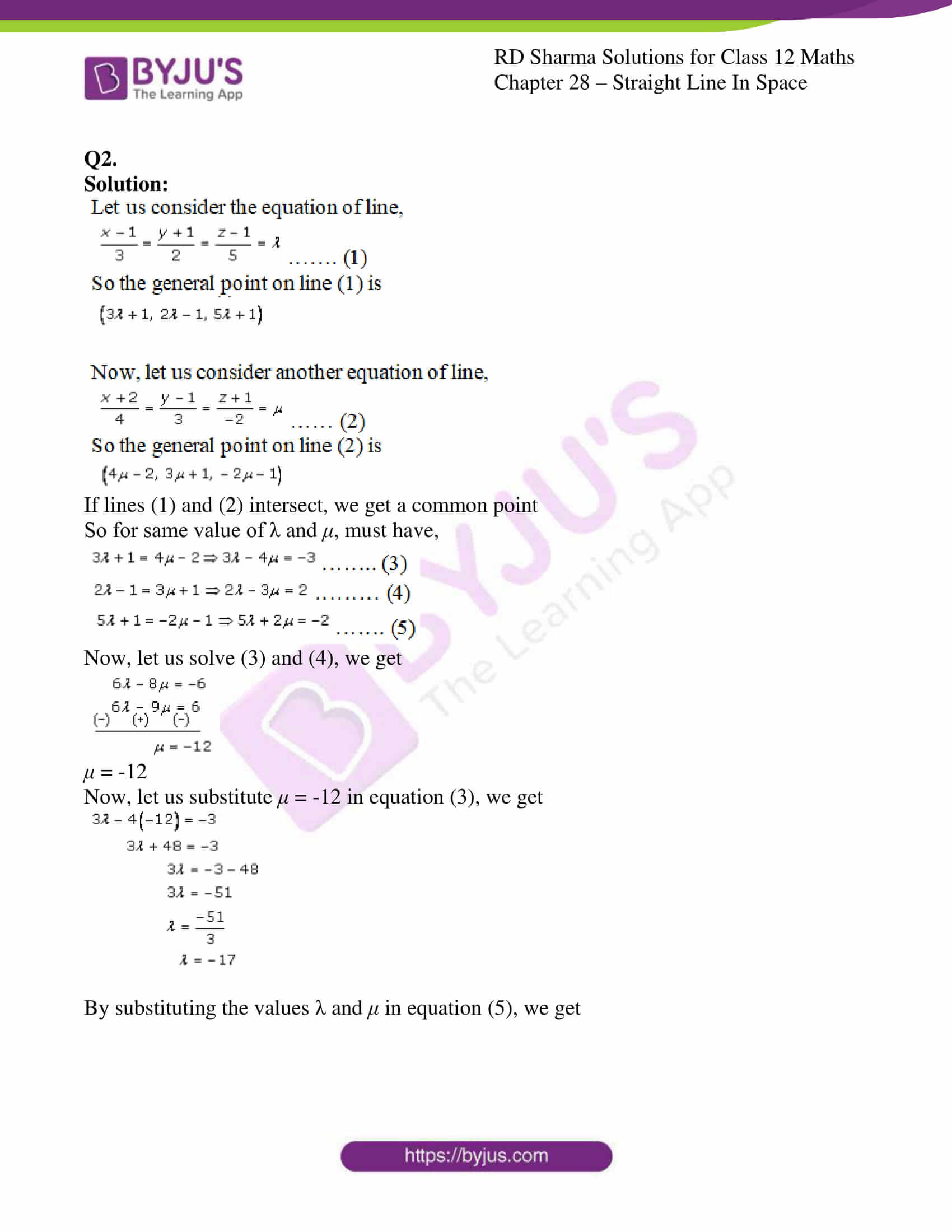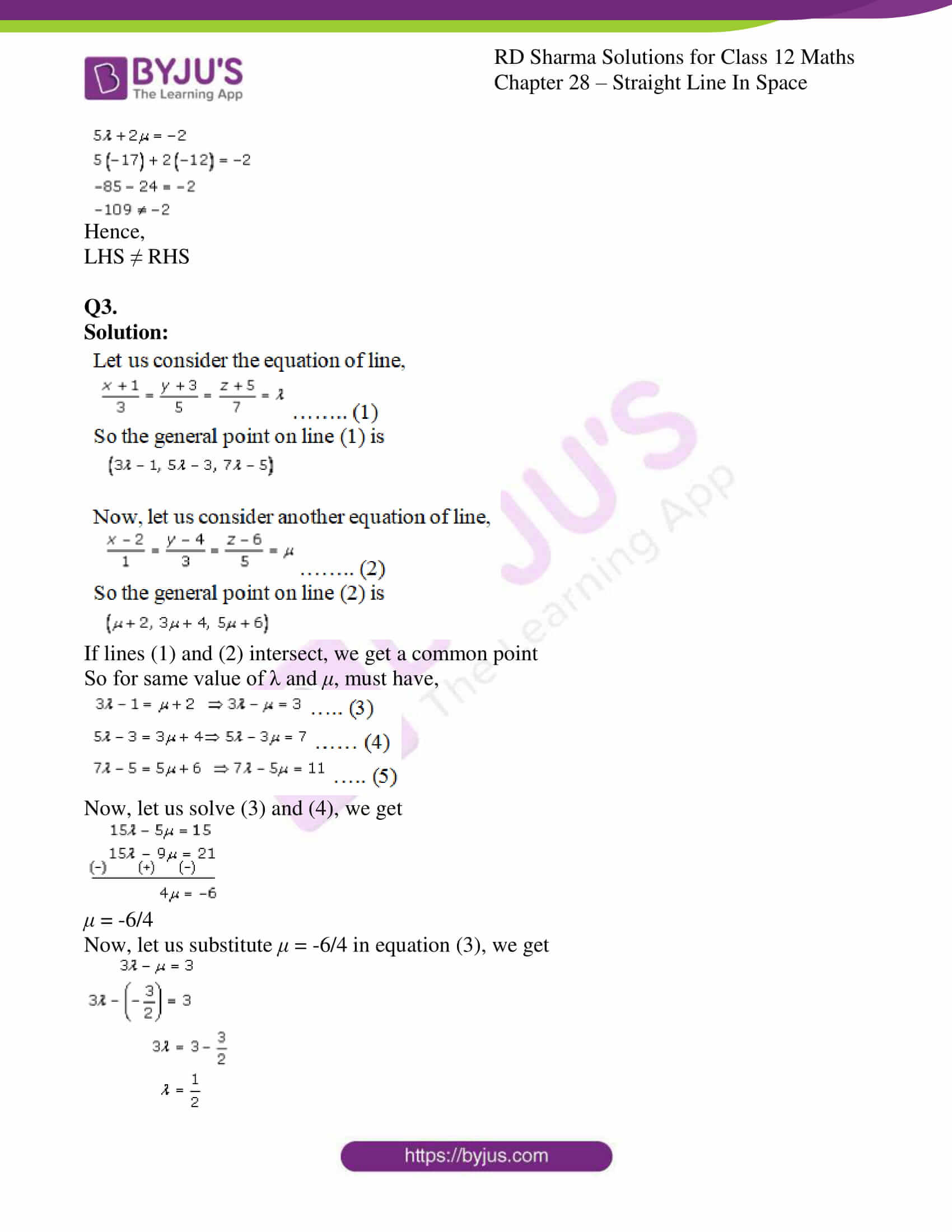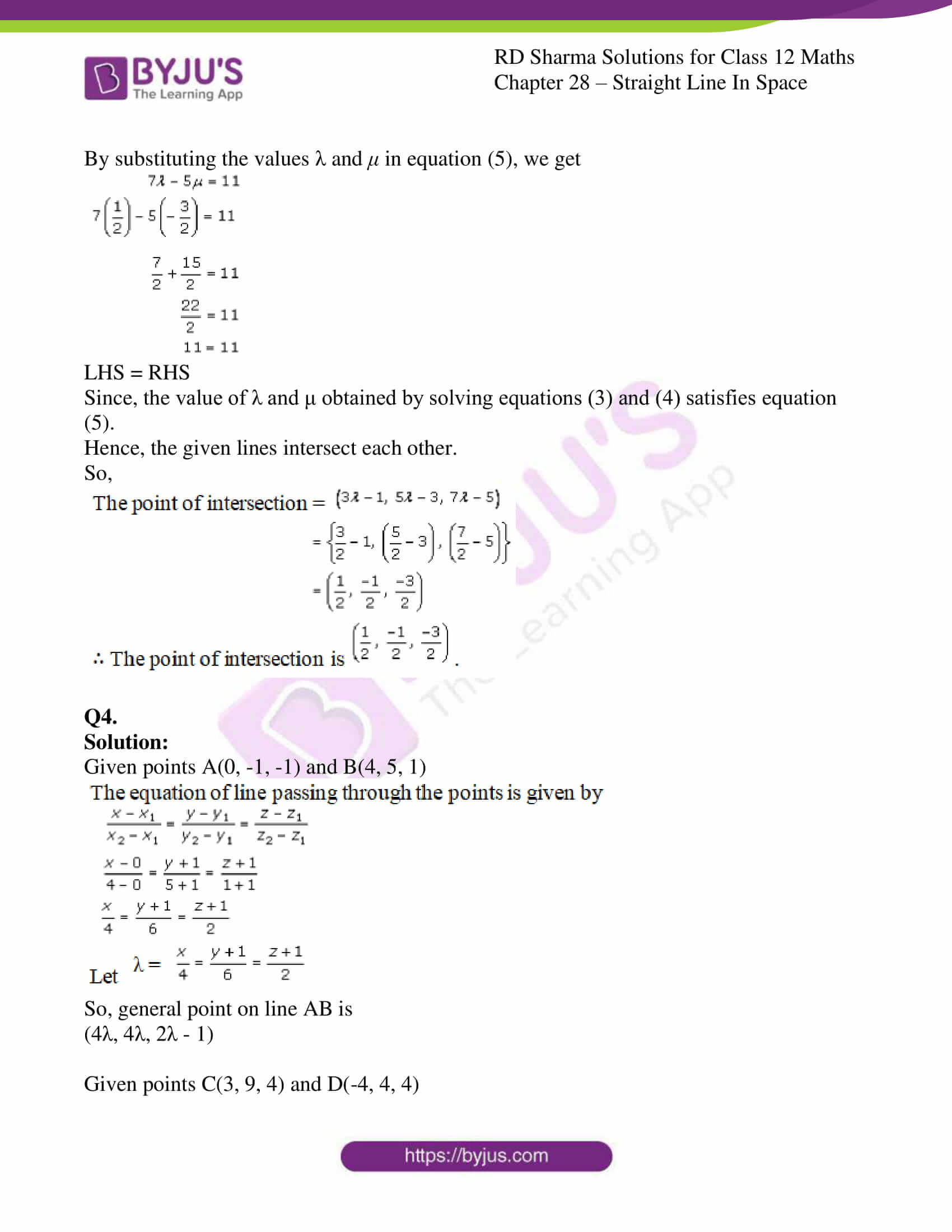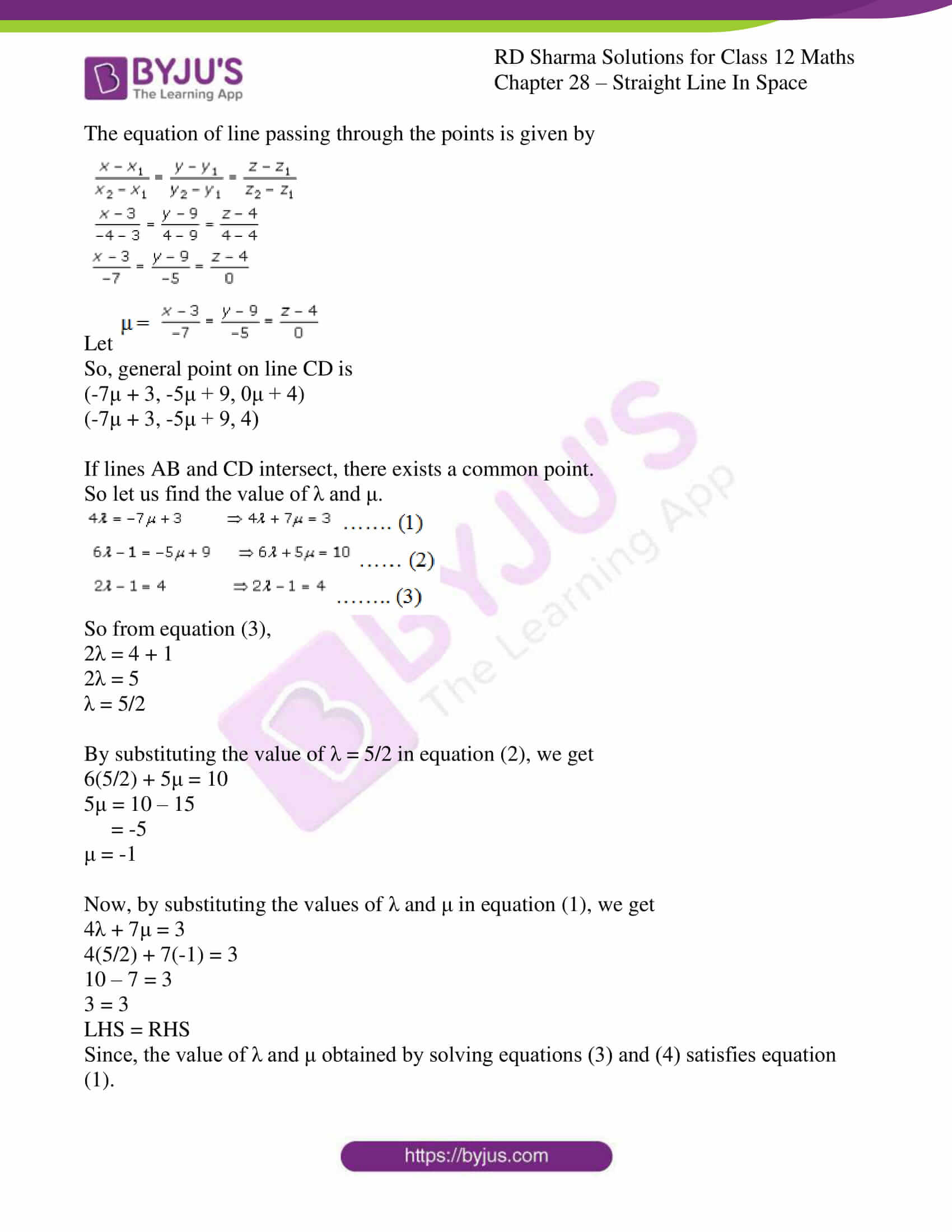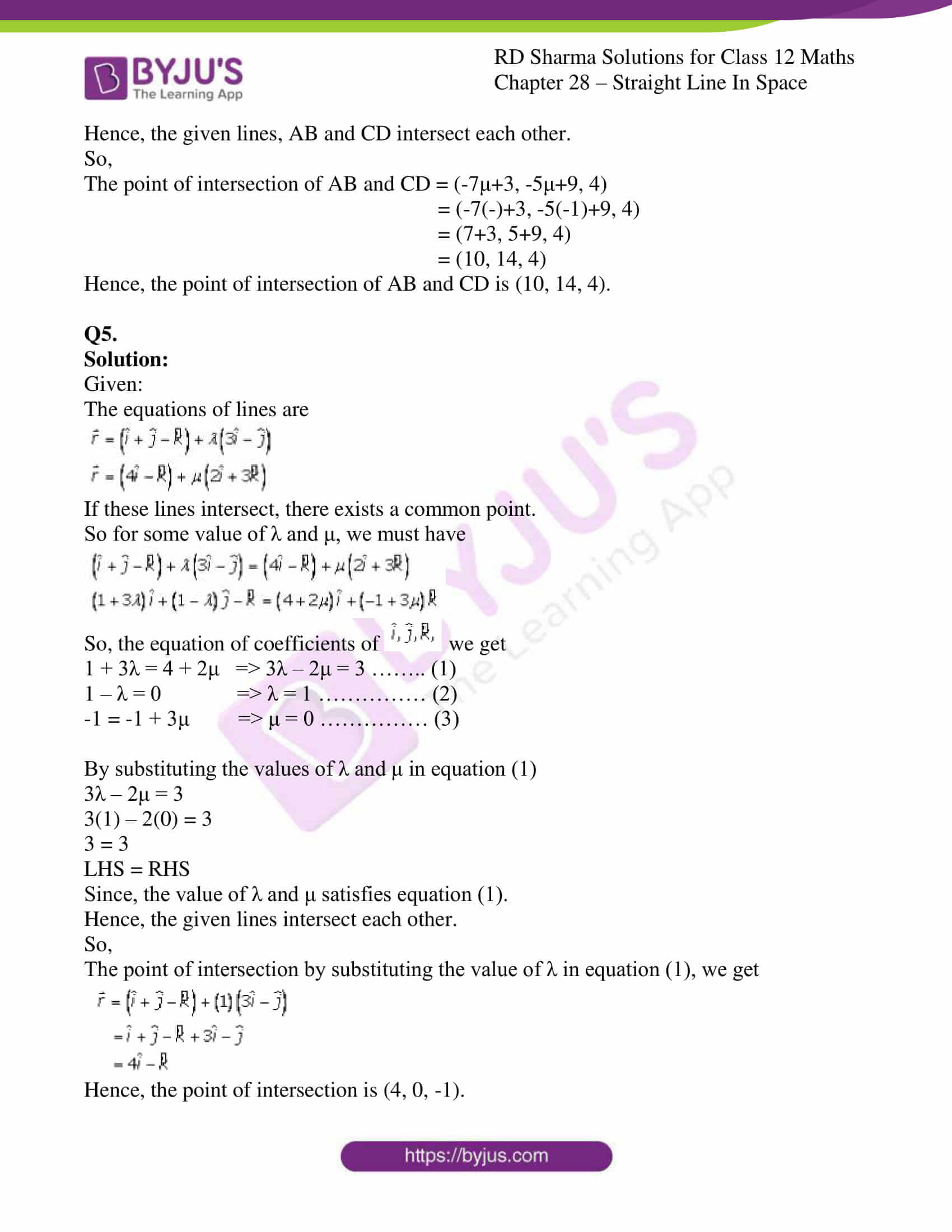### Access Answers for Rd Sharma Solution Class 12 Maths Chapter 28 Exercise 3

Q1.

Solution:

Let us consider the equation of line,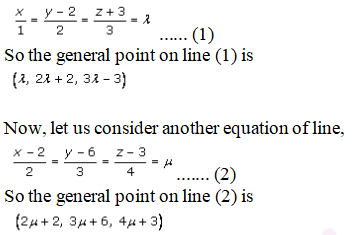If lines (1) and (2) intersect, we get a common point

So for same value of λ and μ, must have,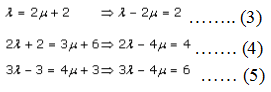Now, let us solve (3) and (4), we get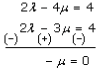μ = 0

Now, let us substitute μ = 0 in equation (3), we get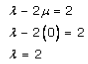By substituting the values λ and μ in equation (5), we get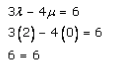Hence,

LHS = RHS

Q2.

Solution: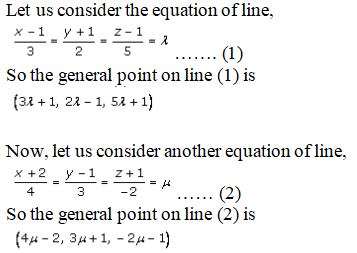If lines (1) and (2) intersect, we get a common point

So for same value of λ and μ, must have,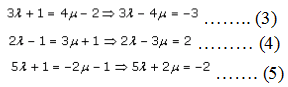Now, let us solve (3) and (4), we get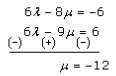μ = -12

Now, let us substitute μ = -12 in equation (3), we get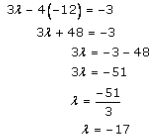By substituting the values λ and μ in equation (5), we get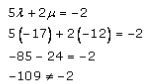Hence,

LHS ≠ RHS

Q3.

Solution: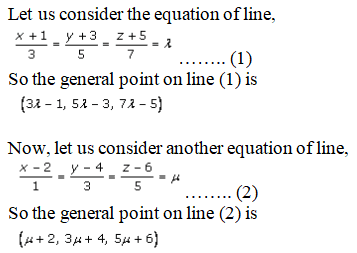If lines (1) and (2) intersect, we get a common point

So for same value of λ and μ, must have,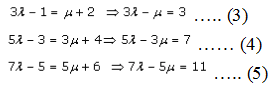Now, let us solve (3) and (4), we get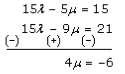μ = -6/4

Now, let us substitute μ = -6/4 in equation (3), we get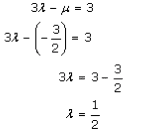By substituting the values λ and μ in equation (5), we get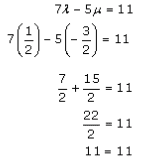LHS = RHS

Since, the value of λ and μ obtained by solving equations (3) and (4) satisfies equation (5).

Hence, the given lines intersect each other.

So,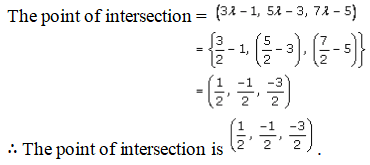Q4.

Solution:

Given points A(0, -1, -1) and B(4, 5, 1)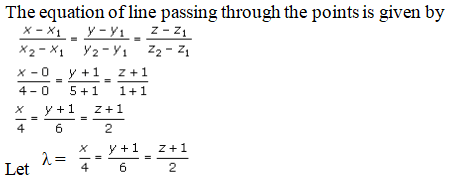So, general point on line AB is

(4λ, 4λ, 2λ – 1)

Given points C(3, 9, 4) and D(-4, 4, 4)

The equation of line passing through the points is given by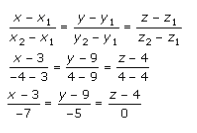Let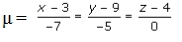So, general point on line CD is

(-7μ + 3, -5μ + 9, 0μ + 4)

(-7μ + 3, -5μ + 9, 4)

If lines AB and CD intersect, there exists a common point.

So let us find the value of λ and μ.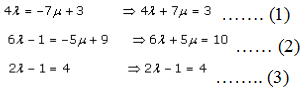So from equation (3),

2λ = 4 + 1

2λ = 5

λ = 5/2

By substituting the value of λ = 5/2 in equation (2), we get

6(5/2) + 5μ = 10

5μ = 10 – 15

= -5

μ = -1

Now, by substituting the values of λ and μ in equation (1), we get

4λ + 7μ = 3

4(5/2) + 7(-1) = 3

10 – 7 = 3

3 = 3

LHS = RHS

Since, the value of λ and μ obtained by solving equations (3) and (4) satisfies equation (1).

Hence, the given lines, AB and CD intersect each other.

So,

The point of intersection of AB and CD = (-7μ+3, -5μ+9, 4)

= (-7(-)+3, -5(-1)+9, 4)

= (7+3, 5+9, 4)

= (10, 14, 4)

Hence, the point of intersection of AB and CD is (10, 14, 4).

Q5.

Solution:

Given:

The equations of lines are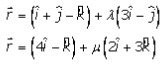If these lines intersect, there exists a common point.

So for some value of λ and μ, we must have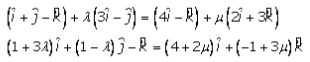So, the equation of coefficients of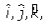we get

1 + 3λ = 4 + 2μ => 3λ – 2μ = 3 …….. (1)

1 – λ = 0 => λ = 1 …………… (2)

-1 = -1 + 3μ => μ = 0 …………… (3)

By substituting the values of λ and μ in equation (1)

3λ – 2μ = 3

3(1) – 2(0) = 3

3 = 3

LHS = RHS

Since, the value of λ and μ satisfies equation (1).

Hence, the given lines intersect each other.

So,

The point of intersection by substituting the value of λ in equation (1), we get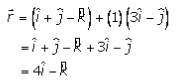Hence, the point of intersection is (4, 0, -1).